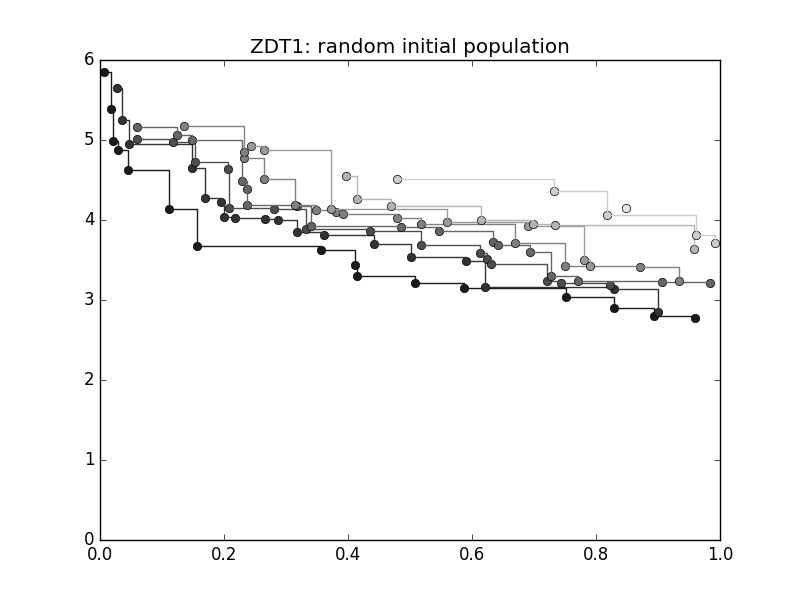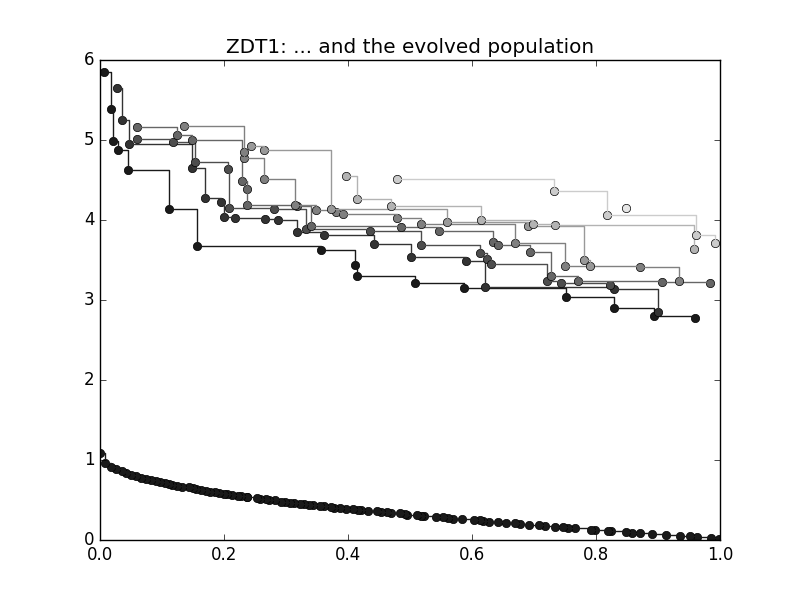# Basic Multi-Objective Functionalities#

In this tutorial we will learn how to use pygmo to solve multiple-objective optimization problems. We assume that you already know how to write your own multi-objective problem (UDP) and will thus here focus on some added functionalities that are intended to facilitate the analysis of your problem.

Let us start to define our population:

```>>> from pygmo import *
>>> udp = zdt(prob_id = 1)
>>> pop = population(prob = udp, size = 10, seed = 3453412)
```

We here make use of first problem of the ZDT benchmark suite implemented in `zdt` and we create a `population` containing 10 individuals randomly created within the box bounds. Which individuals belong to which non-dominated front? We can immediately see this by running the fast non-dominated sorting algorithm `fast_non_dominated_sorting()`:```>>> ndf, dl, dc, ndl = fast_non_dominated_sorting(pop.get_f())
[array([3, 4, 7, 8, 9], dtype=uint64), array([0, 5, 1, 6], dtype=uint64), array(, dtype=uint64)]
```

A visualization of the different non-dominated fronts can also be easily obtained. For example, generating a new `population` with 100 individuals:

```>>> from matplotlib import pyplot as plt
>>> pop = population(udp, 100)
>>> ax = plot_non_dominated_fronts(pop.get_f())
>>> plt.ylim([0,6])
>>> plt.title("ZDT1: random initial population")
```

where each successive pareto front is plotted in darker colour. If we now type:

```>>> algo = algorithm(moead(gen = 250))
>>> pop = algo.evolve(pop)
>>> ax = plot_non_dominated_fronts(pop.get_f())
>>> plt.title("ZDT1: ... and the evolved population")
```

we have instantiated the algorithm `moead`, able to tackle multi-objective problems, fixing the number of generations to 250. In the following line we use directly the method `pygmo.moead.evolve()` of the algorithm to evolve the `population`

The entire population is now on one non-dominated front as can be easily verified typing:

```>>> ndf, dl, dc, ndl = fast_non_dominated_sorting(pop.get_f())
>>> print(ndf)
[array([ 0,  1,  2,  3,  4,  5,  6,  7,  8,  9, 10, 11, 12, 13, 14, 15, 16,
17, 18, 19, 20, 21, 22, 23, 24, 25, 26, 27, 28, 29, 30, 31, 32, 33,
34, 35, 36, 37, 38, 39, 40, 41, 42, 43, 44, 45, 46, 47, 48, 49, 50,
51, 52, 53, 54, 55, 56, 57, 58, 59, 60, 61, 62, 63, 64, 65, 66, 67,
68, 69, 70, 71, 72, 73, 74, 75, 76, 77, 78, 79, 80, 81, 82, 83, 84,
85, 86, 87, 88, 89, 90, 91, 92, 93, 94, 95, 96, 97, 98, 99], dtype=uint64)]
```

The problems in the `pygmo.zdt` problem suite (as well as those in the `pygmo.dtlz`) have a nice convergence metric implemented called p_distance. We can check how well the non dominated front is covering the known Pareto-front

```>>> udp.p_distance(pop)
0.03926512747685471
```

If we are not happy on the value of such a metric, we can evolve the population for some more generations to improve the figure:

```>>> pop = algo.evolve(pop)
>>> udp.p_distance(pop)
0.010346571321103046
```